Concepts

Class 9
Chapter 11 Class 9 - Work and Energy

Power is

Rate of doing work

Power = Work/Time Taken

P = W/T

Example

Suppose a person does 30 Joules work in 5 Seconds

Calculate Power

Ans

Work = 30 Joules

Time Taken = 5 seconds

Power = Work/Time

P = W/T

P = 30 Joules/5 second

= 6 Joules/second

## What is average power?

Average power is defined as the total work done/total energy consumed divided by the total time taken.

Average power = Total Work done / Total Time

## What is SI Unit of Power?

SI unit of Power is Watt

it is named after famous scientist James Watt

Note

1 Watt = 1 Joule/Second

## What are Other Units of Power

SI unit of Power is Watt but it is also measured in KW,MW,bhp etc

Different Units of Power are

 Name Symbol FORUMULA Watt W 1 Watt = 1 Joule/second Kilowatt KW 1 KW = 1000 W Megawatt MW 1 MW = 10,00,000W Horsepower hp 1 HP = 746W = 0.75KW

## Questions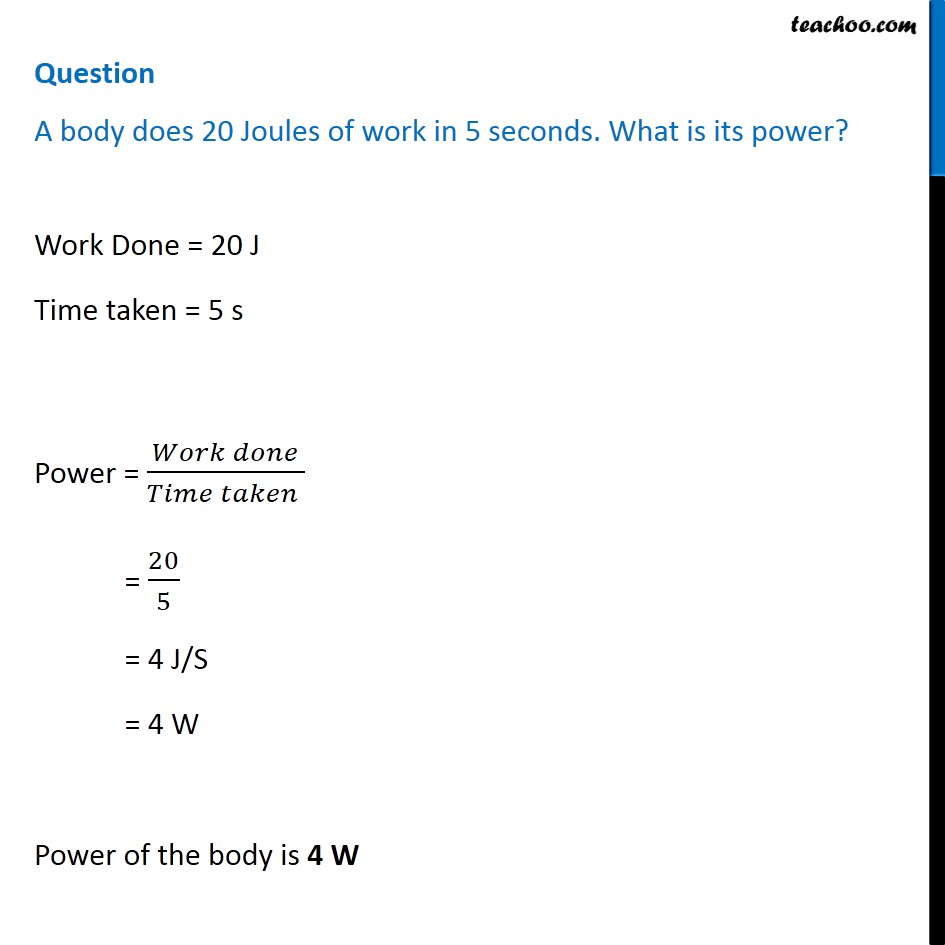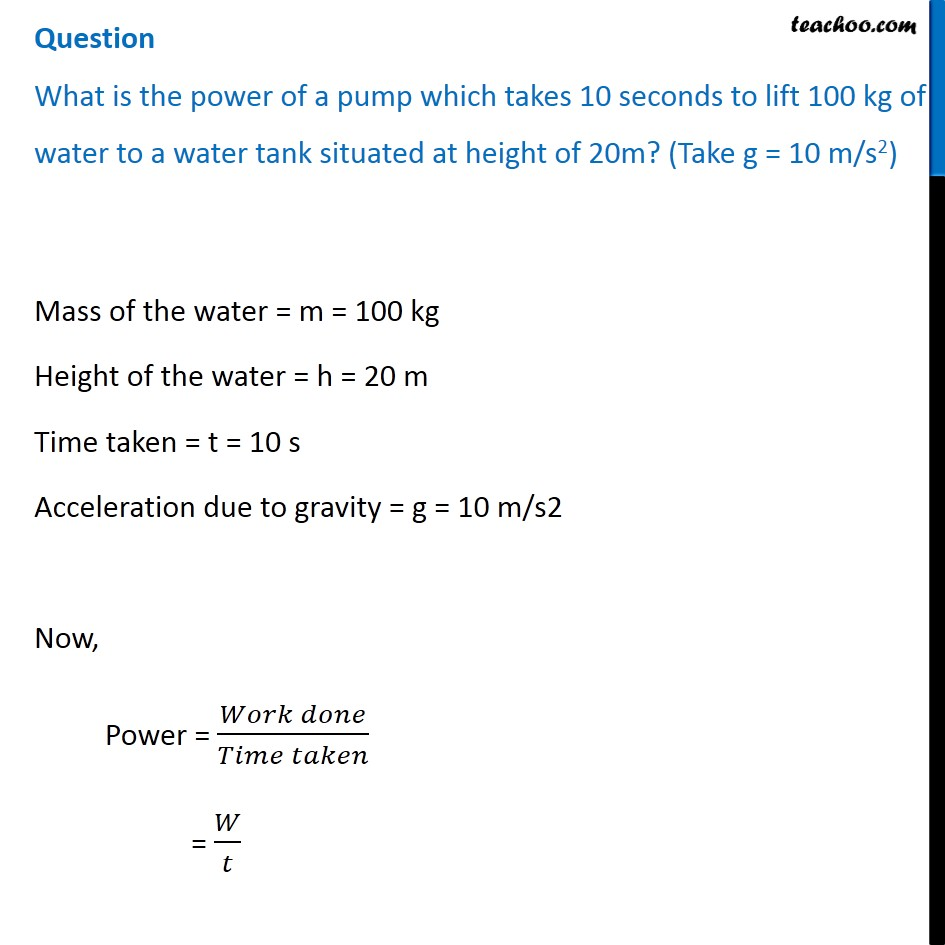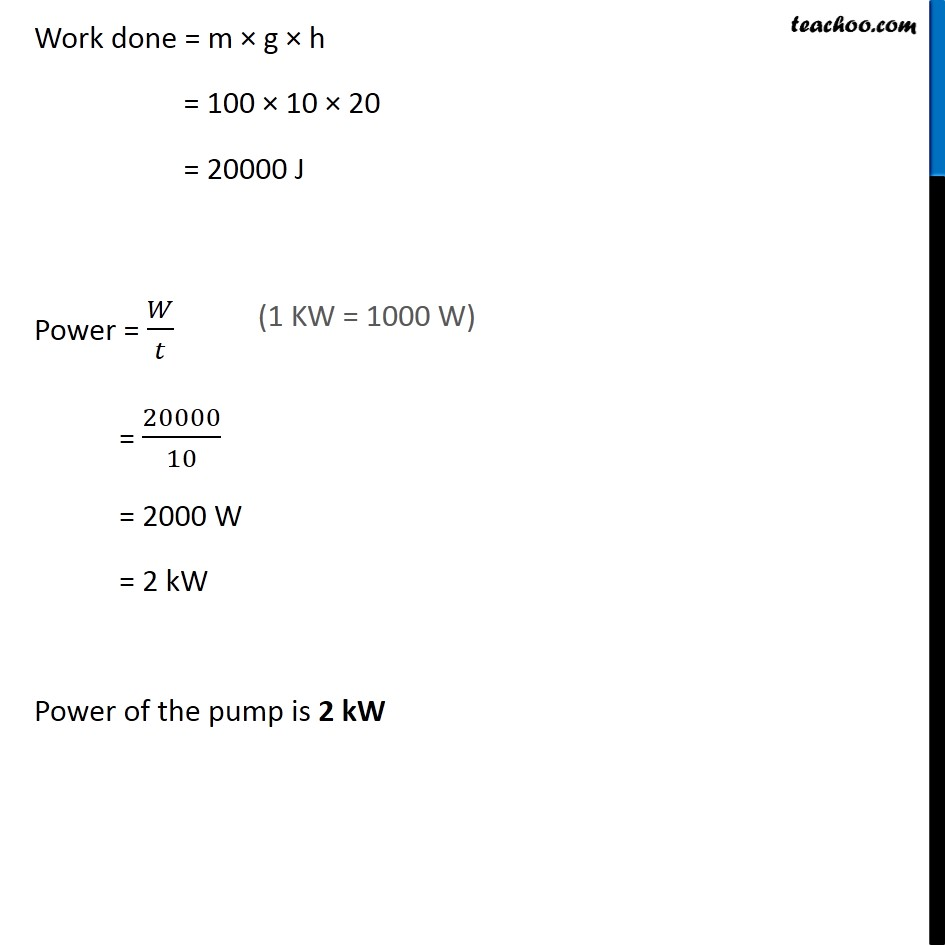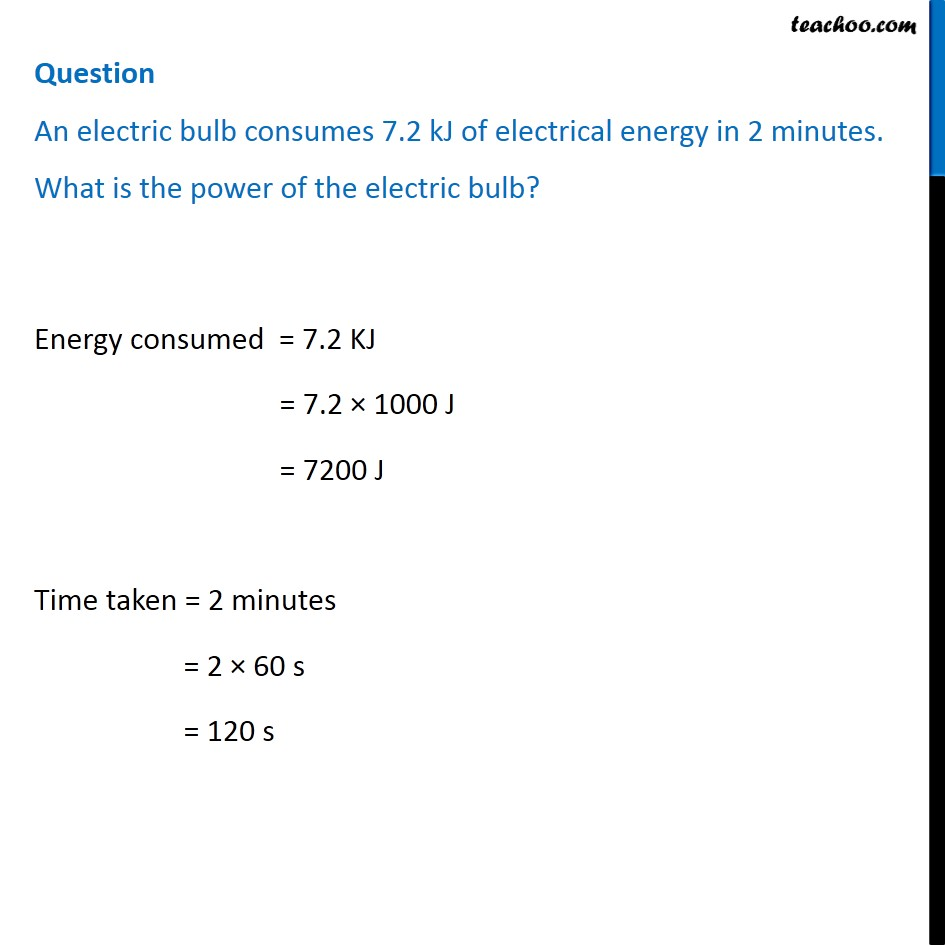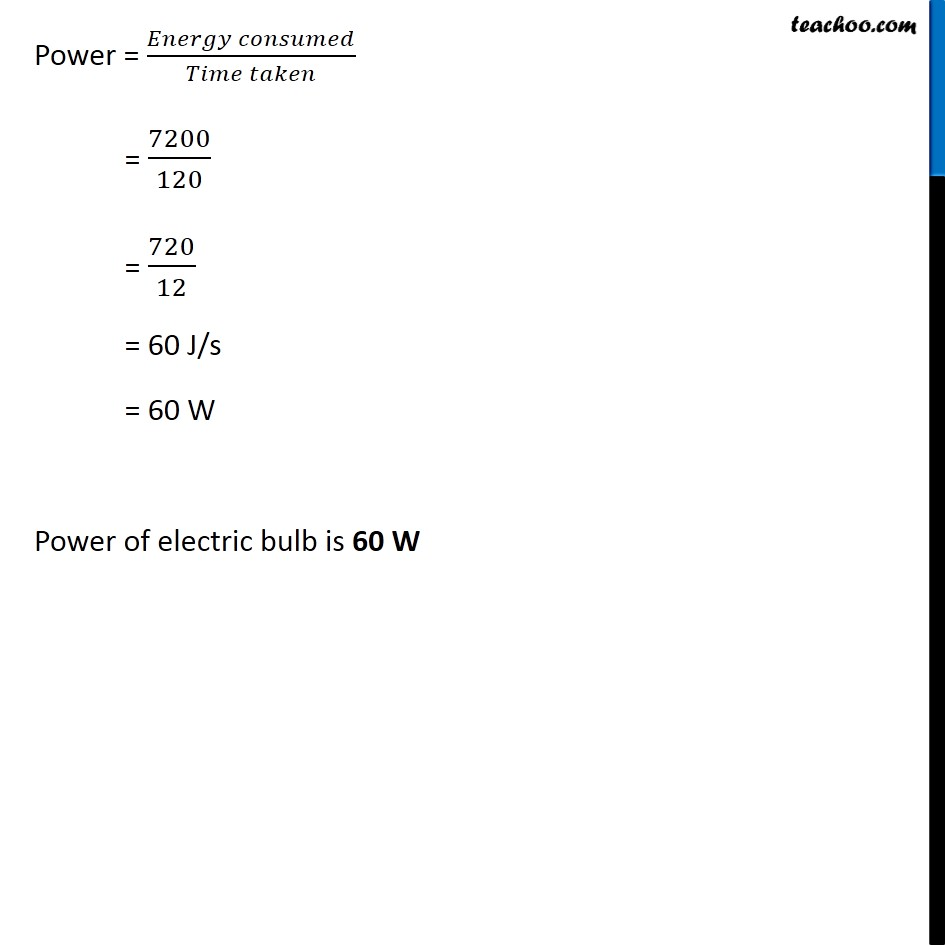Q 1 Page 156 - What is power?

Q 2 Page 156 - Define 1 watt of power

Q 3 Page 156 - A lamp consumes 1000 J of electrical energy in 10 s. What is its power?

Q 4 Page 156 - Define average power

Example 11.7 - Two girls, each of weight 400 N climb up a rope through a height of 8 m. We name one of the girls A and the other B. Girl A takes 20 s while B takes 50 s to accomplish this task. What is the power expended by each girl?

Example 11.8 - A boy of mass 50 kg runs up a staircase of 45 steps in 9 s. If the height of each step is 15 cm, find his power. Take g = 10 m s –2 .

Example 11.9 - An electric bulb of 60 W is used for 6 h per day. Calculate the ‘units’ of energy consumed in one day by the bulb.

NCERT Question 9 - A certain household has consumed 250 units of energy during a month. How much energy is this in joules?

NCERT Question 14 - An electric heater is rated 1500 W. How much energy does it use in 10 hours?

NCERT Question 20 - Find the energy in kWh consumed in 10 hours by four devices of power 500 W each.

Learn in your speed, with individual attention - Teachoo Maths 1-on-1 Class

### Transcript

Question A body does 20 Joules of work in 5 seconds. What is its power? Work Done = 20 J Time taken = 5 s Power = (𝑊𝑜𝑟𝑘 𝑑𝑜𝑛𝑒)/(𝑇𝑖𝑚𝑒 𝑡𝑎𝑘𝑒𝑛 ) = 20/5 = 4 J/S = 4 W Power of the body is 4 W Question What is the power of a pump which takes 10 seconds to lift 100 kg of water to a water tank situated at height of 20m? (Take g = 10 m/s2) Mass of the water = m = 100 kg Height of the water = h = 20 m Time taken = t = 10 s Acceleration due to gravity = g = 10 m/s2 Now, Power = (𝑊𝑜𝑟𝑘 𝑑𝑜𝑛𝑒)/(𝑇𝑖𝑚𝑒 𝑡𝑎𝑘𝑒𝑛) = 𝑊/𝑡 Work done = m × g × h = 100 × 10 × 20 = 20000 J Power = 𝑊/𝑡 = 20000/10 = 2000 W = 2 kW Power of the pump is 2 kW (1 KW = 1000 W) Question An electric bulb consumes 7.2 kJ of electrical energy in 2 minutes. What is the power of the electric bulb? Energy consumed = 7.2 KJ = 7.2 × 1000 J = 7200 J Time taken = 2 minutes = 2 × 60 s = 120 s Power = (𝐸𝑛𝑒𝑟𝑔𝑦 𝑐𝑜𝑛𝑠𝑢𝑚𝑒𝑑)/(𝑇𝑖𝑚𝑒 𝑡𝑎𝑘𝑒𝑛) = 7200/120 = 720/12 = 60 J/s = 60 W Power of electric bulb is 60 W